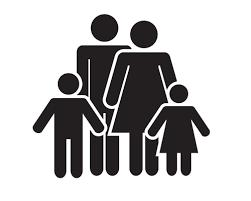# Mother and son

Mother is four times older than her son. In 16 years, the son will be two times younger than his mother. How many years are mother and son?

m =  32
s =  8

### Step-by-step explanation:

m=4s
m+16 = 2·(s+16)

m=4·s
m+16 = 2·(s+16)

m-4s = 0
m-2s = 16

Row 2 - Row 1 → Row 2
m-4s = 0
2s = 16

s = 16/2 = 8
m = 0+4s = 0+4 · 8 = 32

m = 32
s = 8

Our linear equations calculator calculates it.Did you find an error or inaccuracy? Feel free to write us. Thank you!

Tips for related online calculators
Do you have a system of equations and looking for calculator system of linear equations?
Do you want to convert time units like minutes to seconds?